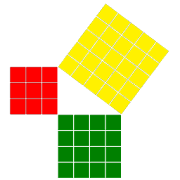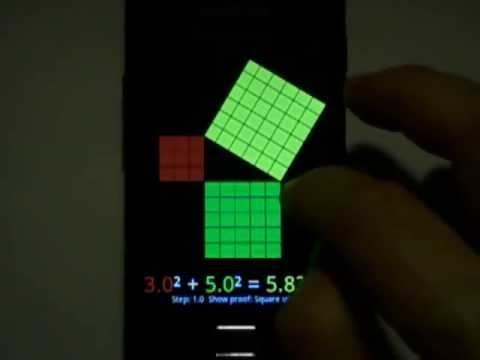# Touch PythagorasEveryone
18The Pythagorean Theorem Interactive: a^2 + b^2 = c^2

App :
change the lengths of the legs (dragging).
change the length of the hypotenuse with two fingers.
zoom (pinch zoom) and rotate the figure (dragging).

There are 6 ways to view the Pythagorean theorem.
- Unit surfaces.
- Two equivalent square containing the same surface.
- The square for each leg in the square of the hypotenuse (Euclid)
- Pingi - Dudeney proof.
- Da Vinci.

Change the precision of the lengths. (In the contextual menu)

This application is also a small laboratory to investigate about the Pythagorean Theorem:
For example, you can experiment easily, looking for the exact solutions of the Pythagorean Theorem:
3² + 4² = 5² is not the only exact solution:

Below 21, there are 3 primitive triples:
3² + 4² = 5²
5² + 12² = 13²
6² + 8² = 10² (Not a true primitive result: Multiple of 3,4,5)
8² + 15² = 17²
9² + 12² = 15² (Not a true primitive result: Multiple of 3,4,5)
12² + 16² = 20² (Not a true primitive result: Multiple of 3,4,5)
Likewise it is also possible to find the solutions below 31 (11 solutions in all: but only 5 primitives)
Or solutions below 101 (52 solutions in all: but only 16 primitives)

More primitive pythagorean triples:
9² + 40² = 41²
11² + 60² = 61²
12² + 35² = 37²
13² + 84² = 85²
15² + 112² = 113²
16² + 63² = 65²
17² + 144² = 145²
19² + 180² = 181²
20² + 21² = 29²
20² + 99² = 101²
24² + 143² = 145²
28² + 45² = 53²
33² + 56² = 65²
36² + 77² = 85²
39² + 80² = 89²
44² + 117² = 125²
48² + 55² = 73²
51² + 140² = 149²
52² + 165² = 173²
57² + 176² = 185²
60² + 91² = 109²
65² + 72² = 97²
85² + 132² = 157²
88² + 105² = 137²
95² + 168² = 193²
104² + 153² = 185²
119² + 120² = 169²
133² + 156² = 205²
140² + 171² = 221²

Touch Math Apps:
http://www.nummolt.com

MathTools classification: http://mathforum.org/mathtools/tool/206418/

M 4 Triangles
M 6 All previous and: Geometry in the plane, Pythagorean theorem
M 7 All previous and: Quadrilateral properties
Geometry All previous Right Triangles, Pythagorean theorem, Trigonometry
Algebra Exponents
Algebra II General
Trigonometry

Alexander Bogomolny: Cut the knot: #112 proofs of the Pythagorean Theorem:
http://www.cut-the-knot.org/pythagoras/
Collapse

Review Policy
4.1
18 total
5
4
3
2
1

## What's New

1.1.6 code 10 - updated to Android Pie after gogle request
1.1.5 Code 9 - minor improvement in contextual menu
1.1.4 Code 8 - new contextual menu
1.1.3 Code 7 - Minor improvement in Euclid proof
Collapse

Updated
October 12, 2019
Size
117k
Installs
5,000+
Current Version
1.1.6
Requires Android
4.0 and up
Content Rating
Everyone
Permissions
Offered By
nummolt
Developer
Maurici Carbó C/ Sant Antoni Maria Claret, 324 Barcelona 08041 SPAIN